# Stochastic Analysis

### V4F1 - Summer semester 2020

Schedule: Tuesday 12.15-14.00 and Thursday 12.15-14.00, (Kleiner Hörsaal, Wegelerstr. 10)  Online until further notice

Tutorial classes: Daria Frolova (Wednesday 16-18, SemR 1.007), Min Liu (Monday 16-18, SemR 1.007) Online until further notice.

Please register in eCampus (link) if you would like to follow the course and the tutorials. Lectures will start the week of April 20th in an online format via Zoom, details on the connections will be shared via eCampus. Possibly the recording of the sessions will be made available offline via eCampus. Tutorials will start the week of April 27th and will also be held online at the scheduled times.

Exam: First oral exam 27/7-1/8. Second oral exam 14/9-25/9. To attend the exam is mandatory to have reached at least half of the total number of points in the exercise sheets.

Sample exam questions (pdf).

### Topics of course

The course aims to develop applications of stochastic calculus to the study of continuous time stochastic processes.

• SDEs: properties, weak/martingale solutions, transformations, large deviations, link with PDEs
• Local times, martingale representation theorem, variational properties of Brownian motion
• Backward SDEs

Note: the introduction to rough paths (Lectures 25-26) will not be part of the exam.

### Prerequisites

Ito calculus for continuous semi-martingales, see  e.g. Prof. Eberle's lecture notes on "Introduction to Stochastic Analysis" (pdf) and my course "Foundations of Stochastic Analysis" from the WS19/20 (link)

### Lecture Notes

The first part of the course will be based on my the notes for the SS17 (link) and Prof. Eberle's lecture notes for Stochastic Analysis SS16 (pdf) (in particular Chapters 2,3 but excluding processes with jumps). The scripts of the lectures below constitute the main material for preparing the exam.

### Further References

• Rogers/Williams: Diffusions, Markov processes and martingales, Vol.2
• Bass: Stochastic processes
• Protter: Stochastic integration and differential equations

### Problem sheets

• Sheet 0 (discussed in the tutorial) [pdf] (related material can be found in eCampus, in particular the paper of Yamada-Watanabe, Cherny and a note on Osgood's condition)
• Sheet 1 (due 30/4, version 2) [tex|tm|pdf]
• Sheet 2 (due 7/5, version 2) [tex|tm|pdf]
• Sheet 3 (due 14/5, version 2 - some typos corrected) [tex|tm|pdf
• Sheet 4 (due 21/5, version 1) [tex|tm|pdf
• Sheet 5 (due 28/5, version 1) [tex|tm|pdf
• Sheet 6 (due 5/6, version 1) [tex|tm|pdf
• Sheet 7 (due 12/6, version 2) [tex|tm|pdf
• Sheet 8 (due 18/6, version 1) [tex|tm|pdf]
• Sheet 9 (due 26/6, version 1) [tex|tm|pdf]
• Sheet 10 (due 3/7, version 1) [tex|tm|pdf]

### Course Journal

• Lecture 1 (21/4) Introduction. SDEs, weak/strong solutions, uniqueness in law, strong uniquenss [script]
• Lecture 2 (23/4) Relations between notions of uniqueness and existence, various examples. Yamada-Watanabe theorem with proof. [script]
• Lecture 3 (28/4) A theorem of Cherny about Uniqueness of the joint law (X,B) for a weak solution and the "dual" Yadama-Watanabe theorem, proof (to be finished) [script]
• Lecure 4 (30/4) End of the proof of Cherny's theorem. Uniqueness in law + strong sol implies pathwise uniqueness. Levy's caracterisation of multidimensional BM. Ortogonal transformations of BM. Bessel process. Discussion of pathwise uniqueness for SDEs. [script
• Lecture 5 (5/5) Martingale problems, formulation, relation with weak solutions, some properties, time change for martingale problems [script]
• Lecture 6 (7/5) DDS Brownian motion, applications of time-change [script]
• Lecture 7 (12/5) Uniqueness of martingale problems [script]
• Lecture 8 (14/5) One dimensional diffusions [script]
• Lecture 9 (19/5) Girsanov's theorem [script]
• Lecture 10 (21/5) Doob's h-transform and conditioning of diffusions [script]
• Lecture 11 (26/5) Conditioning a Brownian motion to stay positive [script]
• Lecture 12 (28/5) Conditioning a diffusion to never exit a domain [script]
• Lecture 13 (2/6) (not recorded) Novikov condition, change of drift in SDEs [script
• Lecture 14 (4/6) Uniqueness in law via Girsanov's theorem, path integral formula, sampling of diffusions via the path integral, representation of the semigroup of a reversible diffusion with additive noise [script
• Lecture 15 (9/6) Ito-Tanaka formula and local times. [script]
• Lecture 16 (16/6) Regularity of local times, representation of local times [script]
• Lecture 17 (18/6) Local time of Brownian motion, Tanaka's SDE, reflected Brownian motion, relation of local time with supremum, Skorohod lemma. Brownian martingale representation theorem [script]
• Lecture 18 (23/6) Brownian martingale representation theorem. the Markovian proof. [script]
• Lecture 19 (25/6) Entropy on the Wiener space, Boué-Dupuis formula. [script]
• Lecture 20 (30/6) Proof of Boué-Dupuis formula. Application to Gaussian tails of Lipschitz functionals.  [script]
• Lecture 21 (2/7) Large deviations / Laplace principle for families of Brownian functionals. [script]
• Lecture 22 (7/7) Complete the proof of the Laplace principle. Applications to Brownian motion (Schilder's theorem) and to small noise diffusions. [script]
• Lecture 23 (9/7) Introduction to backward SDEs and representation formulas for non-linear PDEs. [script]
• Lecture 24 (14/7) Representation formulas for non-linear PDEs. Introduction to rough paths [script]
• Lecture 25 (16/7) Introduction to rough paths (continued)  [script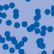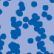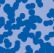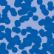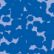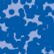## News

Der SFB 1060 Die Mathematik der emergenten Effekte hat eine dritte Förderperiode erhalten. (26.11.20)

Prof. Dr. Andreas Eberle erhält den diesjährigen Lehrpreis der Universität Bonn. (22.07.2020)

Herr Dr. Richard Höfer erhielt den Hausdorff-Gedächtnispreis 2019 der Fachgruppe Mathematik für die beste Disseration. Betreut wurde die Arbeit von Prof. J. Velázquez (29.01.2020).

Einer der Preise der Bonner Mathematischen Gesellschaft (BMG) für die besten Bachelorarbeiten 2019 ging an Herrn Peter Holderrieth unter der Betreuung von Prof. A. Eberle (29.01.2020).

Contact

Managing Director: Prof. Dr. Massimiliano Gubinelli